Squares, roots and powers

This free course is available to start right now. Review the full course description and key learning outcomes and create an account and enrol if you want a free statement of participation.

Free course

2.6.1 Try some yourself

Activity 28

Find each of the following by hand, giving your answers both as a power of ten and as a decimal number. You will use these answers as a check on your calculator work in the next question.

• (a) 10−2

• (b) 102 × 103

• (c) 107 ÷ 104

• (d) 104 ÷ 107

• (e) 2−2

• (a) 10−2 = 0.01

• (b) 102 × 103 = 102 + 3 = 105 = 100 000

• (c) 107 ÷ 104 = 107 − 4 = 103 = 1000

• (d) 104 ÷ 107 = 104 − 7 = 10–3 =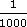= 0.001

• (e) 2−2 =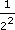=(=0.25)

Activity 29

• (a) 10.3−2 (to four significant figures)

• (b) 10.12 × 10.113 (to four significant figures)

• (c) 10.1127 ÷ 10.214 (to one decimal place)

• (d) 10.124 ÷ 10.3517 (to three significant figures)

• (e) 2.2−2

Using Question 1 as a rough check, the calculator gives:

• (a) 10.3−2 = 0.009 426 (to 4 s.f.)

• (b) 10.12 × 10.113 = 105 400 (to 4 s.f.)

• (c) 10.1127 ÷ 10.214 = 994.8 (to 1 d.p.)

• (d) 10.124 ÷ 10.3517 = 0.000824 (to 3 s.f.)

• (e) 2.2−20.2066

It is a good idea to get in the habit of estimating your answers, even if not specifically asked to do so.

Activity 30

Find each of the following by hand.

• (a) 10240

• (b) 10241

• (c) 5−1

• (d) 10−4

• (a) 10240 = 1

• (b) 10241 = 1024

• (c)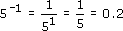• (d)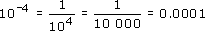Activity 31

How many zeros are there after the decimal point in the number 10−6? How many zeros are there after the decimal point for any given negative power of 10?

10−6 = 0.000 001; there are five zeros after the decimal point. For any given negative power of 10, say 10﻿−(NUMBER) there is one fewer zero than NUMBER. This works for 10−1, too, since 10−1 = 0.1, which has no zeros after the decimal point, i.e. one fewer than 1.

Activity 32

Try to answer the following questions

• (a) Explain why a negative power of a number is one divided by the corresponding positive power of that number. (Hint: remember that a number to the power zero is 1.)

• (b) Use the power button on your calculator to find the following.

• (i)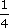• (ii)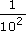• (a) Take an example, which makes the explanation easier than describing it in the abstract. Consider 10−6. Now 6 is just the same as 0 − 6, so 10−6 = 100−6. Using the rule for dividing powers, 100−6 = 100 ÷ 106. But 100 = 1. So 10−6 = 1 ÷ 106. This argument would apply equally well to any number, since any number to the power 0 is 1. (Alternatively you may have started from the other end, for example, by showing that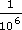=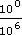= 100−6 = 10−6.)

• (b)

•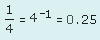•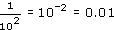MU120_4M4